# Maharashtra Board Class 9 Maths Solutions Chapter 4 Ratio and Proportion Practice Set 4.4

## Maharashtra State Board Class 9 Maths Solutions Chapter 4 Ratio and Proportion Practice Set 4.4

Question 1.
Fill in the blanks of the following.Solution:Question 2.
5m – n = 3m + 4n, then find the values of the following expressions.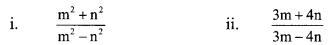Solution:
5m – n = 3m + 4n … [Given]
∴ 5m – 3m = 4n + n
∴ 2m = 5n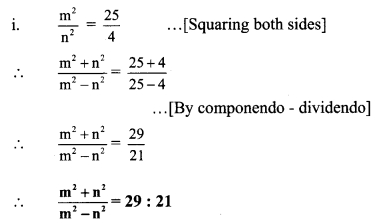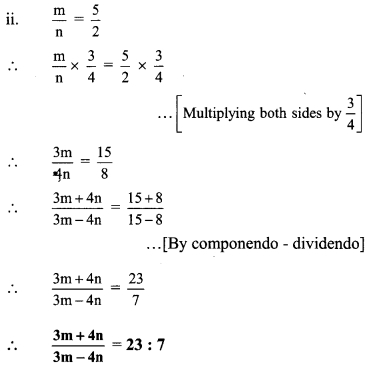Question 3.
Solve:
i. If a(y + z) = b(z + x) = c(x + y) and out of a, b, c no two of them are equal, then show that, $$\frac{y-z}{a(b-c)}=\frac{z-x}{b(c-a)}=\frac{x-y}{c(a-b)}$$.
Solution:
Here, no two of a, b and c are equal.
∴ values of (b – c), (c – a) and (a – b) are not zero.
a(y + z) = b(z + x) = c(x + y) … [Given]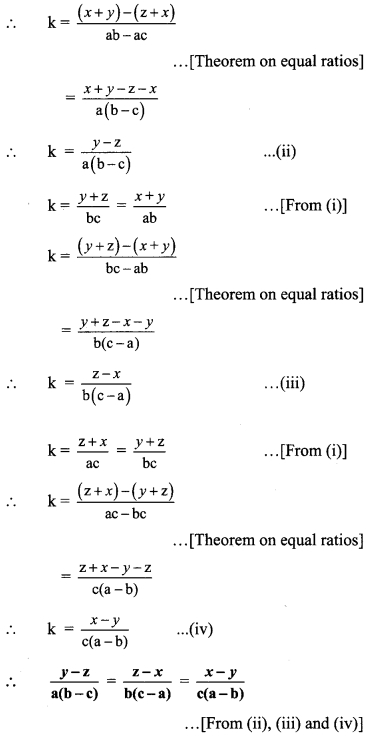ii. If $$\frac{x}{3 x-y-z}=\frac{y}{3 y-z-x}=\frac{z}{3 z-x-y}$$ and x + y + z ≠ 0, then show that the value of each ratio is equal to 1.
Solution: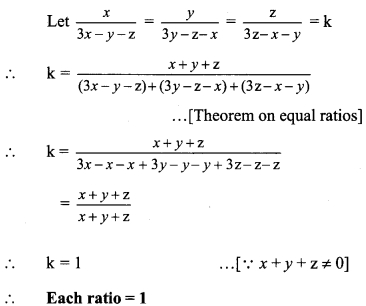iii. $$\frac{x}{3 x-y-z}=\frac{y}{3 y-z-x}=\frac{z}{3 z-x-y}$$ and x + y + z ≠ 0,then show that $$\frac { a+b }{ 2 }$$.
Solution: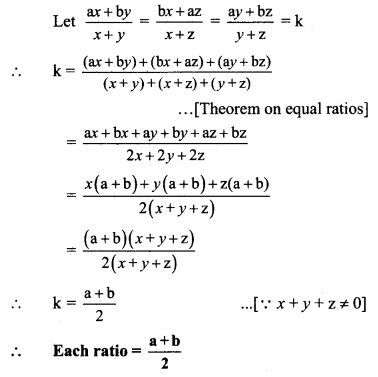iv. If $$\frac{y+z}{a}=\frac{z+x}{b}=\frac{x+y}{c}$$ , then show that $$\frac{x}{b+c-a}=\frac{y}{c+a-b}=\frac{z}{a+b-c}$$.
Solution: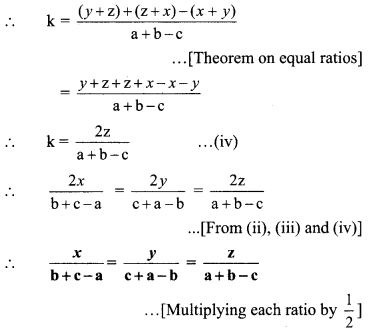v. If $$\frac{3 x-5 y}{5 z+3 y}=\frac{x+5 z}{y-5 x}=\frac{y-z}{x-z}$$ , then show that every ratio = $$\frac { x }{ y }$$.
Solution: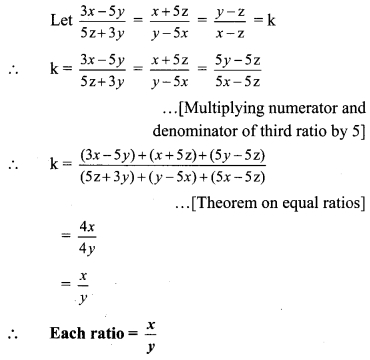Ratio And Proportion Class 9 Practice Set 4.4 Question 4.
Solve: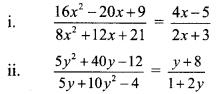Solution:∴ 7(4x – 5)3(2x + 3)
∴ 28x – 35 = 6x + 9
∴ 28x – 6x = 9 + 35
∴ 22x = 44
∴ x = 2
∴ x = 2 is the solution of the given equation.

ii. $$\frac{5 y^{2}+40 y-12}{5 y+10 y^{2}-4}=\frac{y+8}{1+2 y}$$∴ y + 8 = 3(1 + 2y)
∴ y + 8 = 3 + 6y
∴ 8 – 3 = 6y – y
∴ 5 = 5y
∴ y = 1
∴ y = 1 is the solution of the given equation.